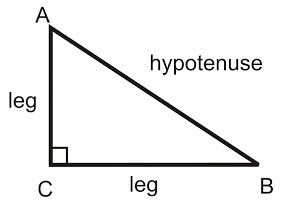Find the hypotenuse of a right triangle with this pythagorean theorem calculator.

### Right Triangle HypotenuseLeg A Length:
Leg B Length:
Fill in the lengths of both legs to determine hypotenuse.

### What is the Hypotenuse?

The hypotenuse is the longest side of a triangle.

What is the Pythagorean Theorem?

Also known as Pythagoras' Theorem, the Pythagorean Theorem is an
equation that solves for the length of the hypotenuse of a right triangle and has some very important uses for us in the real world. Real Life Uses for The Pythagorean Theorem

To calculate the hypotenuse, use the pythagorean theorem as follows:

A2 + B2 = C2

A and B are the lengths of the legs of the triangle. C is the hypotenuse.

Example: A right triangle with a length of Leg A as 50 inches and a
length of Leg B as 50 inches has a hypotenuse of:

502 + 502 = C2

C2 = 5000

C = 70.7107 inches